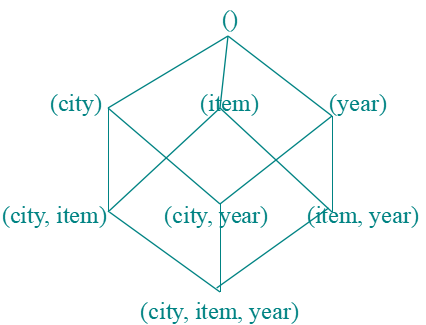# Data Warehouse Implementation - Efficient Data Cube Computation

## Data Warehouse Implementation

The big data which is to be analyzed and handled to draw insights from it will be stored in data warehouses.

These warehouses are run by OLAP servers which require processing of a query with seconds.

So, a data warehouse should need highly efficient cube computation techniques, access methods, and query processing techniques.

The core of multidimensional data analysis is the efficient computation of aggregations across many sets of dimensions.

In SQL aggregations are referred to as group-by’s.

Each group-by can be represented as a cuboid.

Set of group-by’s forms a lattice of a cuboid defining a data cube.

## Efficient Data Cube Computation

The compute cube Operator and the Curse of Dimensionality

The compute cube operator computes aggregates over all subsets of the dimensions specified in the operation.

It requires excessive storage space, especially for a large number of dimensions.

A data cube is a lattice of cuboids.

Suppose that we create a data cube for ProElectronics(Company) sales that contains the following: city, item, year, and sales_in_dollars.

Compute the sum of sales, grouping by city, and item.
Compute the sum of sales, grouping by city.
Compute the sum of sales, grouping by item.

What is the total number of cuboids, or group-by’s, that can be computed for this data cube?

Three attributes:
city, item, year (dimensions), sales_in_dollars (measure).

The total number of cuboids or group-by’s computed for this cube is 2^3=8.

Group-by’s: {(city,item,year), (city, item), (city, year), (item, year), (city), (item), (year),()}.
() : group-by is empty i.e. the dimensions are not grouped.

The base cuboid contains all three dimensions.

Apex cuboid is empty.

On-line analytical processing may need to access different cuboids for different queries.

So we have to compute all or at least some of the cuboids in the data cube in advance.

Precomputation leads to fast response time and avoids some redundant computation.

A major challenge related to precomputation would be storage space if all the cuboids in the data cube are computed, especially when the cube has many dimensions.

The storage requirements are even more excessive when many of the dimensions have associated concept hierarchies, each with multiple levels.

This problem is referred to as the Curse of Dimensionality.

## Cube OperationCube definition and computation in DMQL
• define cube sales_cube[ city, item, year] (sales_in_dollars)
• compute cube sales_cube

Transform it into a SQL-like language (with a new operator cube by, introduced by Gray et al.’96)
• SELECT item, city, year, SUM (amount) FROM SALES CUBE BY item, city, year
Data cube can be viewed as a lattice of cuboids
• The bottom-most cuboid is the base cuboid.
• The top-most cuboid (apex) contains only one cell.
• How many cuboids in an n-dimensional cube with L levels? (T=SUM(Li+1))
• For example, the time dimension as specified above has 4 conceptual levels, or 5 if we include the virtual level all.
• If the cube has 10 dimensions and each dimension has 5 levels (including all), the total number of cuboids that can be generated is 510  9.8x106.

## Data Cube Materialization

There are three choices for data cube materialization given a base cuboid.
• No Materialization
• Full Materialization
• Partial Materialization

How to select which materialization to use
• Identify the subsets of cuboids or subcubes to materialize.
• Exploit the materialized cuboids or subcubes during query processing.
• Efficiently update the materialized cuboids or subcubes during load and refresh.

Selection of which cuboids to materialize
• Based on the size, queries in the workload, accessing cost, their frequencies, etc.

## Indexing OLAP Data: Bitmap Index

First of all, create an index table on a particular column of the table.

Then each value in the column has got a bit vector: bit-op is fast.

The length of the bit vector: # of records in the base table.

The i-th bit is set if the i-th row of the base table has the value for the indexed column.

It's not suitable for high cardinality domains.

## Indexing OLAP Data: Join Indices

The join indexing method gained popularity from its use in relational database query processing.

The join index records can identify joinable tuples without performing costly join operations.

Join indexing is especially useful for maintaining the relationship between a foreign key and its matching primary keys, from the joinable relation.

Suppose that there are 360-time values, 100 items, 50 branches, 30 locations, and 10 million sales tuples in the sales star data cube. If the sales fact table has recorded sales for only 30 items, the remaining 70 items will obviously not participate in joins. If join indices are not used, additional I/Os have to be performed to bring the joining portions of the fact table and dimension tables together.

To further speed up query processing, the join indexing, and bitmap indexing methods can be integrated to form bitmapped join indices.

Microsoft SQL Server and Sybase IQ support bitmap indices. Oracle 8 uses bitmap and join indices.

## Efficient Processing OLAP Queries

The purpose of materializing cuboids and constructing OLAP index structures is to speed up the query processing in data cubes.

Given materialized views, query processing should proceed as follows:

Determine which operations should be performed on the available cuboids:
• Transform drill, roll, etc. into the corresponding SQL and/or OLAP operations, e.g., dice = selection + projection.
Determine to which materialized cuboid(s) the relevant operations should be applied:
• Suppose that the query to be processed be on {brand, province_or_state} with the selection constant “year = 2004”, and there are 4 materialized cuboids available: {year, item_name, city}, {year, brand, country}, {year, brand, province_or_state}, {item_name, province_or_state}  where year = 2004

## Summary

Efficient computation of data cubes:
• Partial vs. full vs. no materialization
• Indexing OALP data: Bitmap index and join index
• OLAP query processing

Subscribe us for more content on Data.